# Getting proper thruster orientation with COM

#### Bingdom

Hello GMC!

I'm currently working on a top-down block physics spaceship prototype. I've run into an issue where I'm having trouble figuring out a great way to activate the correct thrusters to effectively rotate the ship. I've got a working method, but it only works on certain designs.

Here's an example of it not working. (It's meant to be turning clockwise). (Activated thrusters are red). It is trying to rotate the ship 180 degrees away from the mouse.
Note: The little orange arrow in the middle of the ship is the direction the ship is facing.

The centre of mass is located here (The orange circle):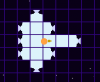I'm not exactly sure what's causing the incorrect rotation. It could be the heavier side having more thrusters.

Another example. This one is meant to be turning clockwise, but it's turning the opposite way. (COM is at the centre)

An example of it working

Why did I show you multiple designs? I do believe it's got something to do with my code and how it behaves.

This is the code I'm using to calculate the direction of the thruster.
Code:
``````with(oThruster) {
var h, v,
//Calculate coordinates for the centre of mass
com_x=other.x+other.com_Px, com_y=other.y+other.com_Py;

//Now calculate how far away the thruster is from the centre of mass.
h=(x-com_x); //Horizontal axis
v=(y-com_y);

//Find out which one of the thruster's axis is closer to the COM
if abs(h) < abs(v) {
//Closer to the COM on the x axis
ang=sign(h*v);
} else {
//Closest on y axis
ang=sign(v*-h);
}
//-1 for turning left (CCW), 1 for turning right (CW) (Works with angle_difference(phy_rotation,-point_dir(x,y,mouse_x/y)

}``````
The system I'm using is that thrusters either have a -1 or 1 value (Turn either left or right). The main block compares the angle between where the whole ship is facing, and the angle from it's location to the mouse. If the result is 1, thrusters that contain a value of 1 will activate, same with thrusters if they had a value of -1.
I am pretty sure the code behind that is fine, just assigning the correct direction of the thrusters is a problem.

Probably not a great system, as some thrusters will be better off not running 100% thrust due to the COM.

Last edited:

#### Bingdom

Perhaps it would be easier to understand if I put some numbers on it.

The green line is the target direction. 0 means the thruster takes no part of rotating the ship. Orange arrow is where the ship is facing.
Working: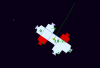Not working:• How do I correctly find what thrusters should thrust to effectively turn the ship towards the target direction, while taking COM into account?
• How can I work out how much thrust the thruster should provide if the ship isn't symmetrical?

#### Matt Hawkins

##### Member
This looks like it would be a lot easier to do if you used box2d physics local force

#### Bingdom

This looks like it would be a lot easier to do if you used box2d physics local force
That's exactly what I'm doing to the thrusters, but that's not the problem.The whole ship composes of physics objects, with adjacent blocks attached as a prismatic joint.

#### Matt Hawkins

##### Member
Ok i seeIt looks like you're having each thruster make its own decision. Is that right? If i was making this I'd control all the thrusters from one object and use variables to turn and move. Say like -
Code:
``````if angle_difference(ang1, ang2) > 0
{
left = true;
}
if left = true
{
thruster1 = true
thruster6 = true
}``````

#### Bingdom

That's similar to what I'm doing, but the problem is determining if the thruster is a "left" or is it a "right" thruster.

The user has the ability to create custom ships. It would be a tedious task assigning the arrangement for all the thrusters.

#### Matt Hawkins

##### Member
Hmm that's a tricky one. You could just have a selection of thrusters that are tied to different inputs.

#### IndianaBones

##### Member
Now this is an interesting problem! Is it Captain Forever inspired by the way?I'm not sure if this is a complete solution, but on paper it works for your ship designs above.

Are you familiar with the dot product operation for two vectors?

It basically tells you how much one vector has in common with another vector.

Or in physics, how much work is being done along a vector based on the force applied by another vector.

Basically:

A positive result means the angle between the two vectors is less than 90 degrees.

A result of 0 means the two vectors are at right angles to each other.

A negative result means the angle between the two vectors is greater than 90 degrees.

Possible solution:

1 ) Calculate the vector(light blue) between the ship current facing direction(pink vector) and the direction you desire to move/rotate to(green vector)Now that we have the blue vector, which is basically the direction we want the ship to rotate in:

(2) For all thrusters that are in front of the centre of mass (relative to the facing), calculate the dot product of the force of the thruster with the vector from step (1) (blue vector)

For example, the yellow vector of the thruster on the left, dot product with the blue vector: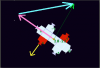For the thrusters that are in front of the center of mass, if the result is negative(less than zero), then activate that thruster, and you can use the result of the dot product to scale how much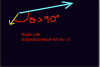Counter example, for a thruster that should not be activated: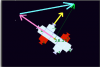(Dot product is positive, DONT activate)

(3) Do the same for all thrusters behind the centre of mass, calculate the dot product, EXCEPT, for these ones, if the result is positive, activate the thruster.

There may be some edge cases, like if the dot product is 0, do you activate the thrusters, in theory I think yes, but you may have to test that.

Anyone who is better at vector maths and/or physics please feel free to correct me if I made a mistake.

References:
https://www.mathsisfun.com/algebra/vectors-dot-product.html
https://www.mathsisfun.com/algebra/vector-calculator.html

•Bingdom

#### Bingdom

Is it Captain Forever inspired by the way
I got the idea from Reassembly.Possible Solution
Thank you for your solution. I managed to implement what you have suggested. However, after implementing it, I ran into a problem. Using your method, thrusters facing a different way (not 180 away from each other) will active at different times. (Likely due to the orientation of the thrusters. Things like 90+180)

For my original method, it was using angle_difference to determine which direction to go.
Code:
``with(thruster) if sign(angle_difference) == ang {thruster turn on}``
(Look at OP to see how ang was obtained)

When you say "all thrusters behind the centre of mass", This is the problem I had.

Turns out, I think I might've solved the problem myself. I did a stress test ship and it looks ok so far. Will need to test it with a few other designs.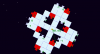For my second question, I do believe I can just use my movement script to counteract the steering script. (WIll look into that once I've found the time)

Last edited:

#### HammerOn

##### Member
(Note that angles are inverted compared to GM in the coordinate system I'm using here)
How do I correctly find what thrusters should thrust to effectively turn the ship towards the target direction, while taking COM into account?
torque = offset x force ("x" means cross product) https://en.wikipedia.org/wiki/Torque
If torque = 0, it can't rotate the ship. If torque < 0 it rotates CCW. If torque > 0 it rotates CW.

All blocks with a circle contribute to CCW rotation:How can I work out how much thrust the thruster should provide if the ship isn't symmetrical?
As for the theory: Each side of the ship needs to apply the same amount of force to make it rotate around the center without displacement. In the image above, for example, the thruster from the right should provide as much torque as all the thrusters from the left. You can distribute the total torque of the side across all the thrusts in that side and rearrange the torque formula to solve for force.

But it's nearly impossible to predict the variables of a complex system like these ships. The constraints add even more complexity to it. We can guess but the range of error is too wide for practical use.
In the real world, most of the machines work by having a target, querying its current state with sensors, and operating its components to correct the current state until it reaches its target. We can say the right approach for automation isn't about predicting what it takes to do a task but to make corrections until it does it.
If you want it to be stable and easy to code, you have to simplify it. Use what I explained for visuals like thruster animation but behind the scenes, the whole ship is just a single primitive shape the same way a complex character with limbs, torso, and many details is just a rectangle for the collision system.

Last edited:
•Bingdom